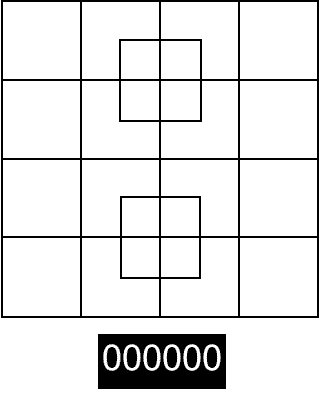Categories

# Count Squares Puzzle

Can you count how many squares are in this picture?Once you have counted all the squares then use comment section and let us know how many squares you could find in this pic. You can match your answer with the one we have published at the end of this post.

If you liked this puzzle then I am sure you would like Puzzle – How many square are there?

Also try: How many Triangles?

Here is answer for count square puzzle:

Answer count the number of squares is 40.

Big block 1

Small blocks 16

The center squares 2

Inside the center squares 8

2×2 block squares 9

3×3 block squares 4There is other simple way to count squares in N x N grid. Just use formula as explained in post How to count squares in N x N grid? .

Also Try: Count Triangles in This Cat – Puzzle

Search Keywords:

Square counting puzzle, square counting riddle, square counting brainteasers, count number of square puzzle, count number of square puzzle answer, square counting answer, Count squares in this picture puzzle